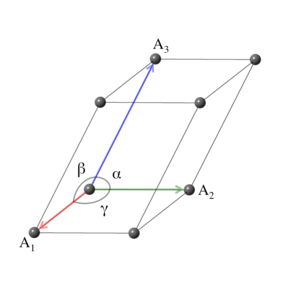# Encyclopedia of Crystallographic Prototypes

M. J. Mehl, D. Hicks, C. Toher, O. Levy, R. M. Hanson, G. L. W. Hart, and S. Curtarolo, The AFLOW Library of Crystallographic Prototypes: Part 1, Comp. Mat. Sci. 136, S1-S828 (2017). (doi=10.1016/j.commatsci.2017.01.017)
D. Hicks, M. J. Mehl, E. Gossett, C. Toher, O. Levy, R. M. Hanson, G. L. W. Hart, and S. Curtarolo, The AFLOW Library of Crystallographic Prototypes: Part 2, Comp. Mat. Sci. 161, S1-S1011 (2019). (doi=10.1016/j.commatsci.2018.10.043)

## The Triclinic Crystal SystemTriclinic is the most general crystal system. All other crystal systems can be considered special cases of the triclinic. The primitive vectors are also completely general: their lengths ($a$, $b$, $c$) and angles ($\alpha$, $\beta$, $\gamma$) may have arbitrary values. The triclinic system has one Bravais lattice, which is also the conventional lattice for this system.

### Lattice 1: TriclinicThere are many choices for the primitive vectors in the triclinic system. We make the choice $\begin{eqnarray} \mathbf{a}_1 & = & a \, \mathbf{\hat{x}} \nonumber \\ \mathbf{a}_2 & = & b \, \cos\gamma \, \mathbf{\hat{x}} + b \, \sin\gamma \,\mathbf{\hat{y}} \nonumber \\ \mathbf{a}_3 & = & c_x \, \mathbf{\hat{x}} + c_y \, \mathbf{\hat{y}} + c_z \, \mathbf{\hat{z}}, \end{eqnarray}$ where $\begin{eqnarray*} c_x & = & c \, \cos\beta \\ c_y & = & \frac{c \, (\cos\alpha - \cos\beta \, \cos\gamma)}{\sin\gamma} \end{eqnarray*}$ and $\begin{eqnarray*} c_z & = & \sqrt{c^2 - c_x^2 - c_y^2}. \end{eqnarray*}$
The volume of the triclinic unit cell is $\begin{equation} V = a \, b \, c_z \, \sin\gamma. \end{equation}$ The space groups associated with the triclinic lattice are \begin{array}{ll} 1. ~ \mbox{P1} & 2. ~ \mbox{P}\overline{1} \\ \end{array}